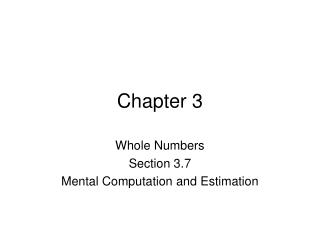DownloadDownload PresentationChapter 3

# Chapter 3

Télécharger la présentation## Chapter 3

- - - - - - - - - - - - - - - - - - - - - - - - - - - E N D - - - - - - - - - - - - - - - - - - - - - - - - - - -
##### Presentation Transcript

1. Chapter 3 Whole Numbers Section 3.7 Mental Computation and Estimation

2. Mental Computation vs Estimation Mental Computation (or computation) is used to find the exact answer to an arithmetic problem. While estimation does not necessarily give the exact answer but an approximate answer. Mental Computation usually refers to being able to get the exact answer to an arithmetic problem in your head without the use of calculator, pencil or paper. There are instances when this is necessary. It is usually with smaller numbers where the arithmetic is straight forward. Mental Addition 1. Break apart: 53 + 49 think of 50+40 and 3+9 gives 90+12 = 102 2. Compensation (Nearest Ten): 53 + 49 think of 52 + 50 gives 102 Mental Subtraction 1. Break apart: 74 – 58 think of 74 – 50 gives 24 and 24 – 8 = 16 2. Compensation: 74 – 58 think of 76 – 60 gives 16 Mental Multiplication 1. Break apart: 56  7 think of 50  7 + 6  7 gives 350 + 42 = 392

3. Estimation This is used to give an approximate answer to some arithmetic problem. We use the symbol “≈” to mean the answer we give is close to the correct answer but not exact. Rounding Strategy The most common rounding strategy is known as a basic fact estimation. You round the numbers you want to add, subtract or multiply to the nearest whole numbers that uses a single digit other than zero. You do the arithmetic with using only the basic whole number arithmetic facts. Examples: A school has 32 students in Kindergarten, 39 in first grade, 41 in second grade and 24 in third grade. Estimate how many seats they will need for a program for K through 3. Rounding each number: 32+39+41+24 ≈ 30+40+40+20 = 130. A teacher wants to buy calculators for each student in her class to use. She has 22 students and each calculator costs \$12.99 each. Use a basic fact approximation to estimate the cost of the calculators. Answer: We need to estimate 22  12.99, rounding each number gives the following: 22  12.99 ≈ 20  10 = 200.

4. Basic Fact Range Estimate Sometimes it is useful to get either the smallest possible or largest possible estimation for an answer. This type is called a range estimate. To find the largest possible basic fact (sometimes called an upper estimate) estimate we round the numbers up to the next biggest digit, to find the smallest (sometimes called a lower estimate) we round down to the next smallest digit. Example A teacher wants to take her class of 24 students to a science museum that charges \$6.99 for each student. Find an upper and lower basic fact estimate for the cost of taking the students to the science museum. Basic Fact Upper estimate for 24  6.99 ≈ 30  7 = 210 Basic Fact Lower estimate for 24  6.99 ≈ 20  6 = 120 The actual cost is between 120 and 210 dollars. Compatible Numbers This type of estimation is commonly used to estimate division problems. You use the numbers that are close to what you want to find to make the problem easier to do. The following is an example using division.

5. Example A school has 348 third grade students they want to take on a field trip. The school can get 9 buses to use. Use a compatible numbers strategy to estimate the number of students on each bus. We want to estimate 348  9 ≈ 360  9 = 40. We use the number 360 instead of 348 because we know 36  9 = 4 so that makes 360 the closest number that is compatible with the division. Front-End Strategy This method just uses the first digit of each number without trying to round it off or make it fit the problem like the two we have talked about. Example A front-end strategy to estimate: 267 + 381 + 5285 + 37 ≈ 200 + 300 + 5000 + 30 = 5530 (The actual answer is: 5970)